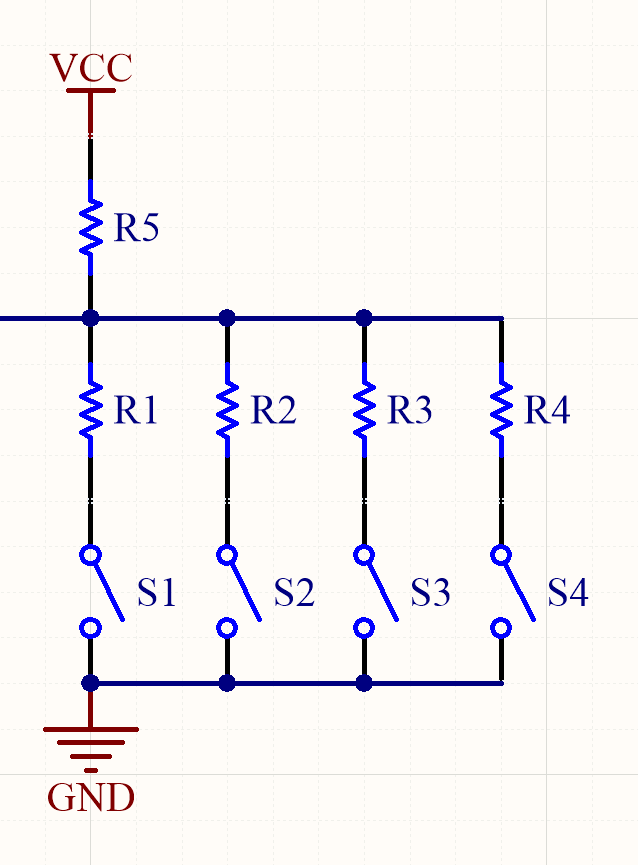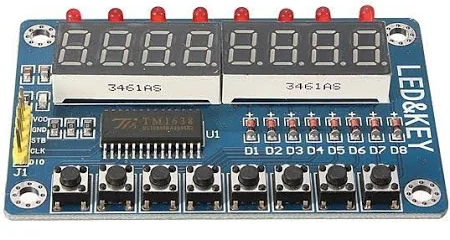jerry_fuyi

AVR单片机教程——矩阵键盘

矩阵键盘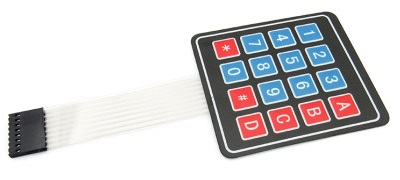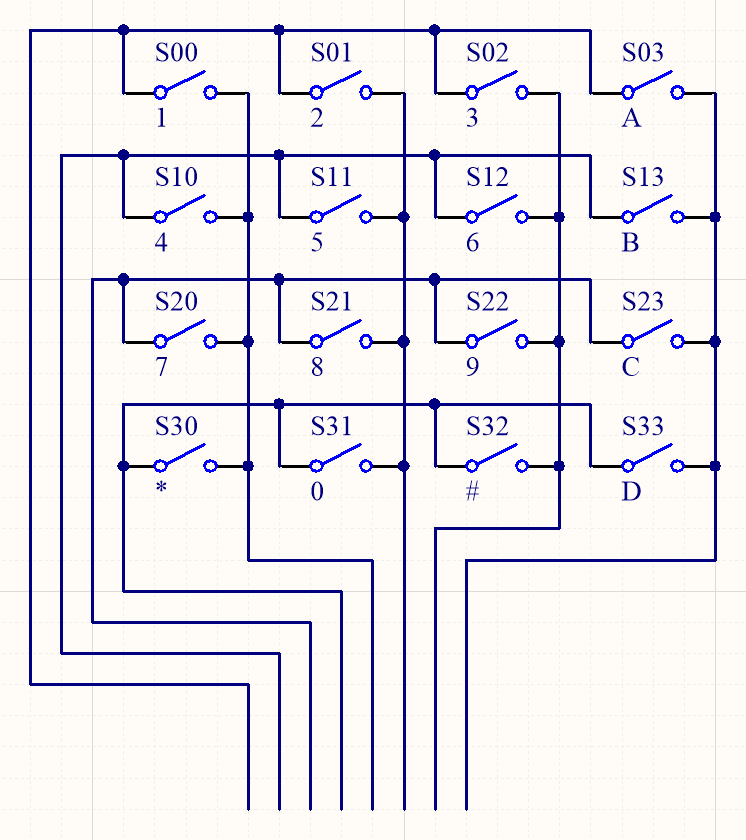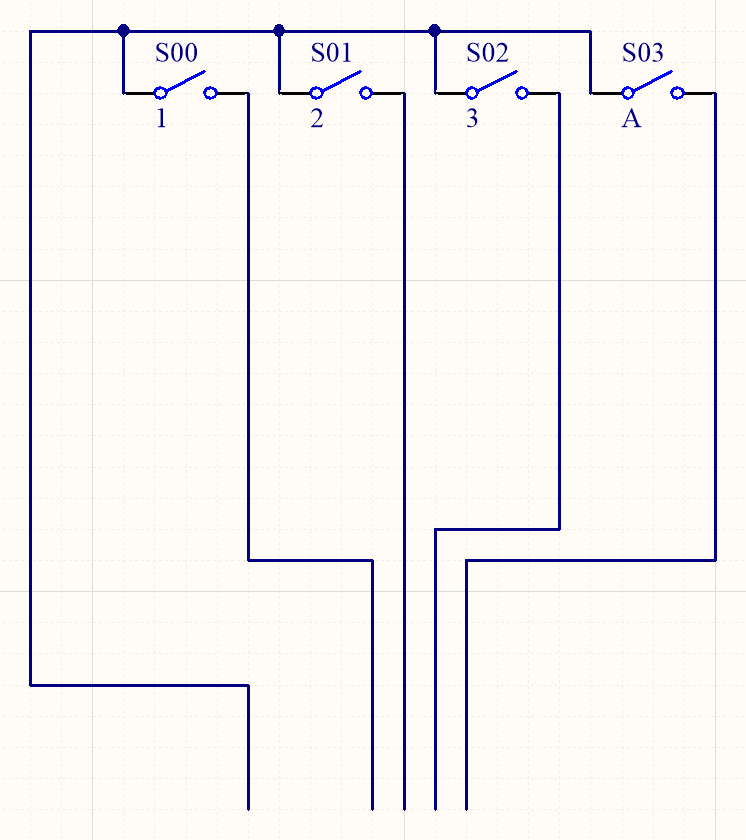#include <ee2/pin.h>
#include <ee2/delay.h>
#include <ee2/uart.h>

int main(void)
{
const pin_t row = {PIN_0, PIN_1, PIN_2, PIN_3};
const pin_t col = {PIN_4, PIN_5, PIN_6, PIN_7};
const char name = {
'1', '2', '3', 'A',
'4', '5', '6', 'B',
'7', '8', '9', 'C',
'*', '0', '#', 'D',
};
bool status = {false};
uart_init(UART_TX_64, 384);
for (uint8_t j = 0; j != 4; ++j)
pin_write(col[j], PULLUP);
while (1)
{
for (uint8_t i = 0; i != 4; ++i)
{
pin_write(row[i], LOW);
pin_mode(row[i], OUTPUT);
for (uint8_t j = 0; j != 4; ++j)
{
uint8_t index = i * 4 + j;
if (status[index] && !cur)
{
uart_print_char(name[index]);
uart_print_line();
}
status[index] = cur;
}
pin_mode(row[i], INPUT);
}
delay(1);
}
}

74HC165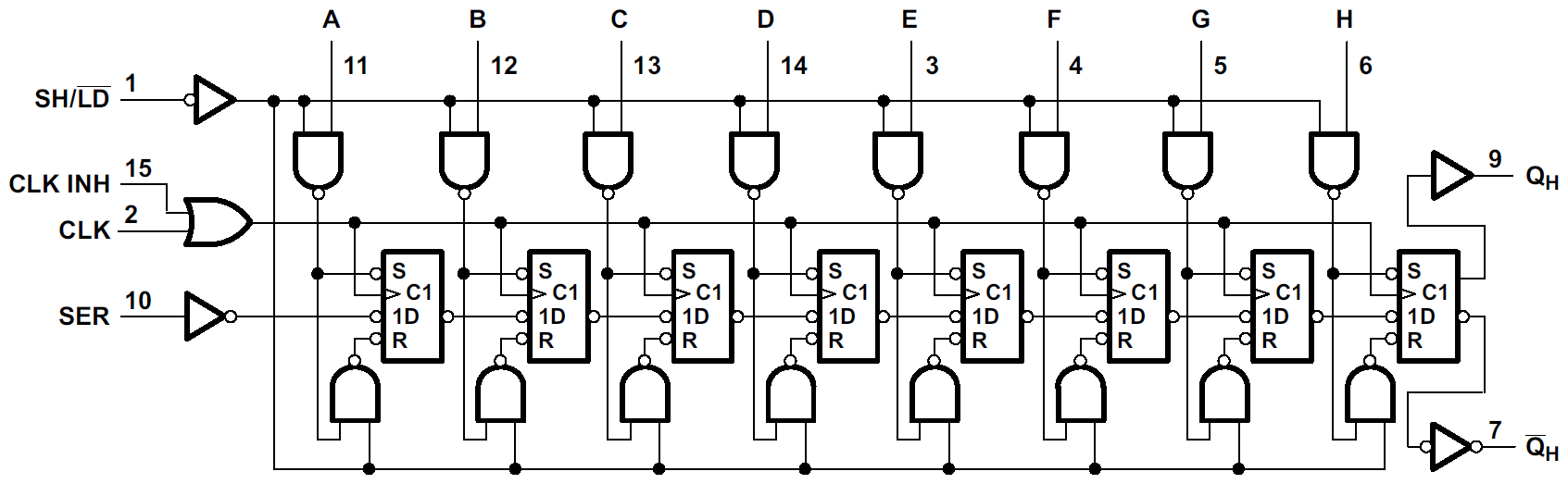SH/LD引脚用于选择移位寄存器的工作模式。当SH/LD为高时，非门输出低，两个与非门一定输出高，D触发器的SR前有个圆圈，表示低电平有效，SR不起作用，移位寄存器在时钟上升沿移位；当SH/LD为低时，非门输出高，两个与非门的输出是另一个输入取非，当A为高和低时分别有SR为低，并行端口上的数据被锁存进移位寄存器中。

{
DDRC  |=   1 << DDC2;    // PC2 output
DDRC  |=   1 << DDC3;    // PC3 output
DDRD  &= ~(1 << DDD2);   // QH  input
DDRD  |=   1 << DDD4;    // CLK output
PORTC |=   1 << PORTC2;  // PC2 high
PORTC |=   1 << PORTC3;  // PC3 high, SH/LD low
PORTC &= ~(1 << PORTC3); // PC3 low,  SH/LD high
PORTD &= ~(1 << PORTD4); // CLK low
uint8_t result = 0;
for (uint8_t i = 0; i != 8; ++i)
{
result >>= 1;            // the bit read first is LSB
if (PIND & (1 << PIND2)) // QH high
result |= 1 << 7;    // set result's MSB
PORTD |=   1 << PORTD4;  // CLK high
PORTD &= ~(1 << PORTD4); // CLK low
}
return result;
}

74HC595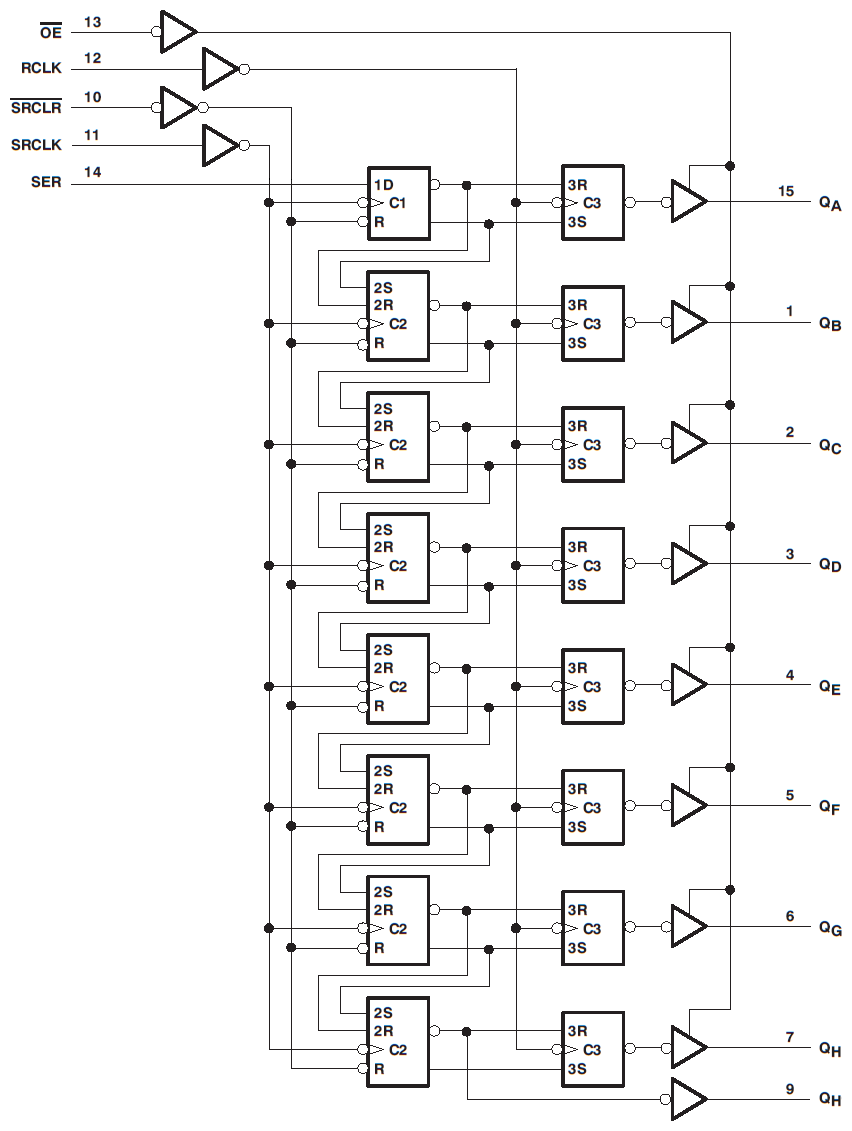595和165是兄弟芯片，结构与165对称。SER为串行输入，8位移位寄存器由时钟信号SRCLK的上升沿控制；RCLK上升沿控制一组RS锁存器，将移位寄存器中的数据反映到QAQH引脚的电平上来；SRCLR低电平有效，异步地将移位寄存器中的数据全部清零；略有不同的是输出级，595支持三态输出，当OE为高电平时高阻输出。

void write_595(uint8_t _data)
{
DDRD  |=    1 << DDD3;    // SER   output
DDRD  |=    1 << DDD4;    // SRCLK output
DDRC  |= 0b11 << DDC2;    // PC3:2 output
PORTD &=  ~(1 << PORTD4); // SRCLK low
for (uint8_t i = 0; i != 8; ++i)
{
if (_data & 1 << 0)          // LSB first
PORTD |=   1 << PORTD3;  // SER high
else
PORTD &= ~(1 << PORTD3); // SER low
_data >>= 1;
PORTD |=   1 << PORTD4;      // SRCLK high
PORTD &= ~(1 << PORTD4);     // SRCLK low
}
#define PC32(x) (PORTC = (PORTC & ~(0b11 << PORTC2)) | (x) << PORTC2)
PC32(0b10); // RCLK low
PC32(0b01); // RCLK high
#undef PC32
}

595最经典的功能就是驱动LED了。事实上，开发板上的数码管和LCD接口都是挂在595的输出上的。现在我们学习了595的用法，终于可以自己点亮数码管了。

#include <ee2/pin.h>
#include <ee2/delay.h>

void write_595(uint8_t _data);

int main()
{
pin_t digit = {PIN_4, PIN_5};
for (uint8_t i = 0; i != 2; ++i)
{
pin_write(digit[i], HIGH);
pin_mode(digit[i], OUTPUT);
}
uint8_t which = {
1, 1, 1, 1, 0, 0, 0, 0
};
uint8_t pattern = {
0b00000001, 0b00000010, 0b00000100, 0b00001000,
0b00001000, 0b00010000, 0b00100000, 0b00000001
};
while (1)
for (uint8_t i = 0; i != 8; ++i)
{
pin_write(digit[which[i]], LOW);
write_595(pattern[i]);
delay(200);
pin_write(digit[which[i]], HIGH);
}
}

595也是支持级联的，方法是多片595共用SRCLKRCLK，一片的QH'连接下一片的SER。但是当级联的595数量很多时，刷新一次输出是比较耗时的，可以考虑换一种组织方式，把一串595换成多组级联，每一组第一个595的SER连接单片机，所有595共用SRCLKRCLK，可以有效减少级联长度。这是用引脚数量换取速度，具体还是应该根据需求来权衡。

综合实践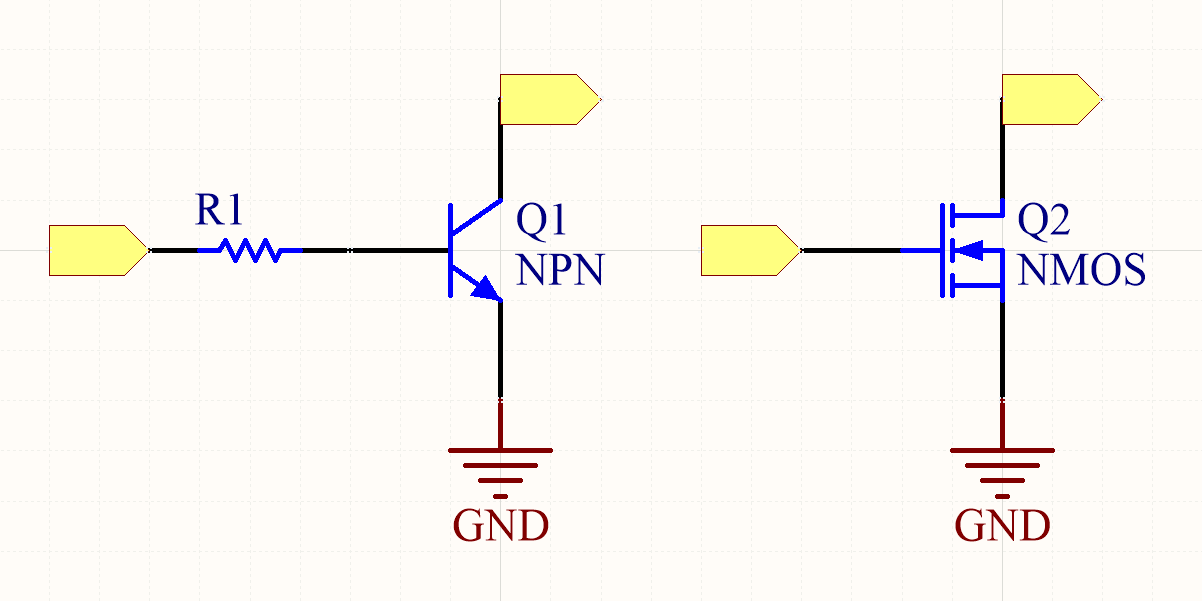• Q1是一个NPN型的三极管，左边的基极（B）串联了电阻后作为输入，下方的发射极（E）接地，上方的集电极（C）作为输出。当输入高电平时，有电流从基极流向发射极，三极管就允许有电流从集电极流向发射极，可以认为输出低电平；当输入低电平时，基极与发射极之间没有电流，集电极与发射极之间也不能有电流，可以认为输出高阻态。

• Q2是一个N沟道的MOS管，左边的栅极（G）作为输入，下方的源极（S）接地，上方的漏极（D）作为输出。当输入高电平时，漏极和源极之间出现导电沟道，并且电阻很小，输出为低电平；当输入低电平时，没有导电沟道，输出为高阻态。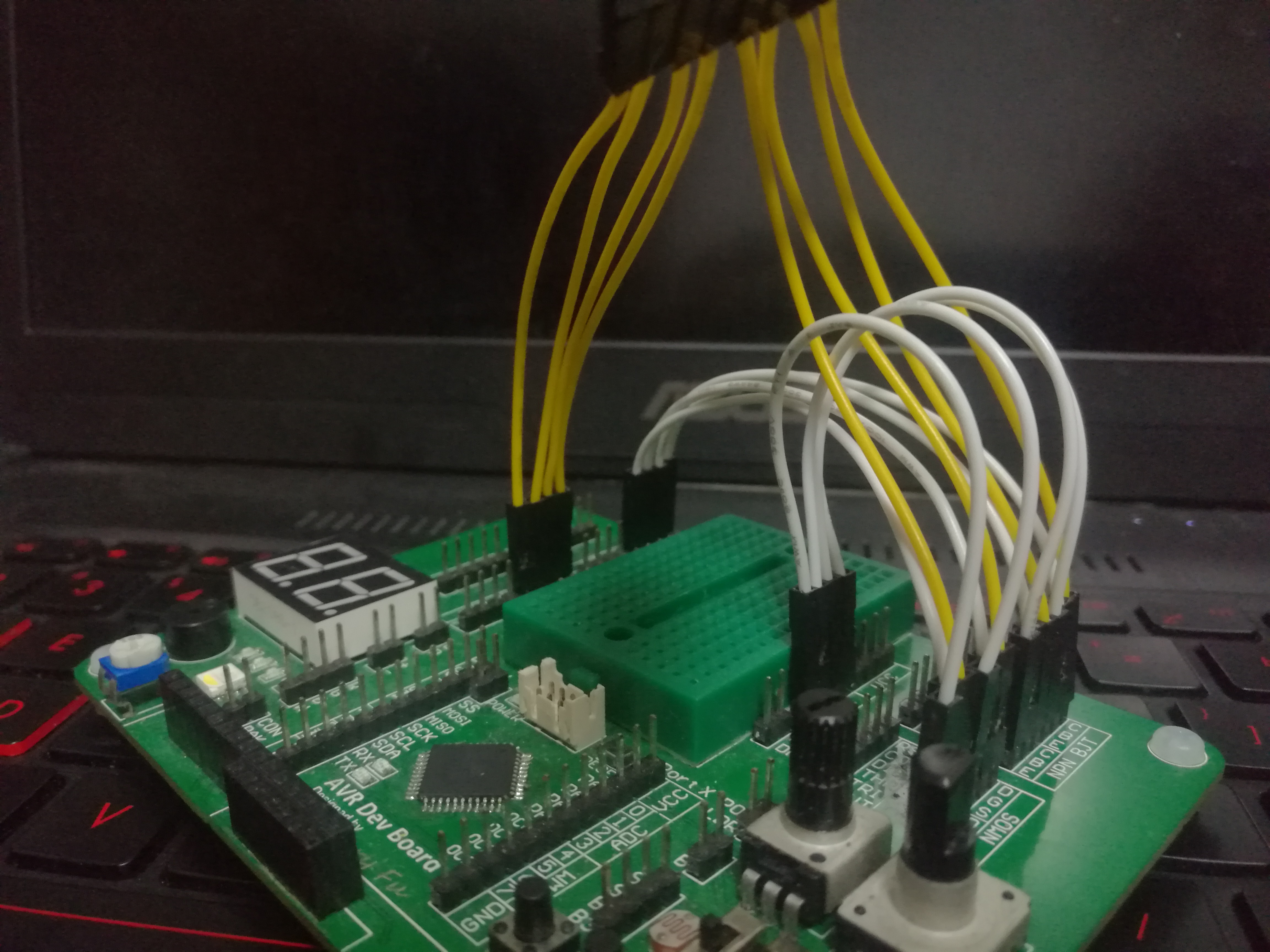#include <ee2/bit.h>
#include <ee2/exout.h>
#include <ee2/exin.h>
#include <ee2/uart.h>
#include <ee2/timer.h>

void timer()
{
static const char name = {
'1', '2', '3', 'A',
'4', '5', '6', 'B',
'7', '8', '9', 'C',
'*', '0', '#', 'D',
};
static bool status = {false};
static uint8_t phase = 0;
if (phase & 1)
{
for (uint8_t i = 0; i != 4; ++i)
{
uint8_t index = (phase >> 1) * 4 + i;
if (status[index] && !cur)
{
uart_print_char(name[index]);
uart_print_line();
}
status[index] = cur;
}
}
else
{
exout_write(1 << (phase >> 1));
}
if (++phase == 8)
phase = 0;
}

int main()
{
exout_init();
exin_init();
uart_init(UART_TX_64, 384);
timer_init();
timer_register(timer);
while (1)
;
}

作业

1. 有时候程序会无缘无故判定出一次按键按下，特别是松开按键的时候，原因是单片机读取到的电平存在抖动。请你解决这个问题。

2. 根据图示习惯，我判断74HC165逻辑图中的D触发器的SR引脚是异步的、电平触发的。请你写程序来验证这个事实。